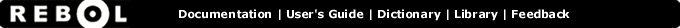# Tangent - Function Summary

## Summary:

Returns the trigonometric tangent in degrees.

tangent value

## Arguments:

value - The value argument. (must be: number)

## Refinements:

/radians - Value is specified in radians.

## Description:

Ratio between the length of the opposite side to the length of the adjacent side of a right triangle.

 ``` print tangent 30 0.577350269189626```

 ``` print tangent/radians 2 * pi 0.0```

## Related:

arccosine - Returns the trigonometric arccosine in degrees.
arcsine - Returns the trigonometric arcsine in degrees.
arctangent - Returns the trigonometric arctangent in degrees.
cosine - Returns the trigonometric cosine in degrees.
tangent - Returns the trigonometric tangent in degrees.